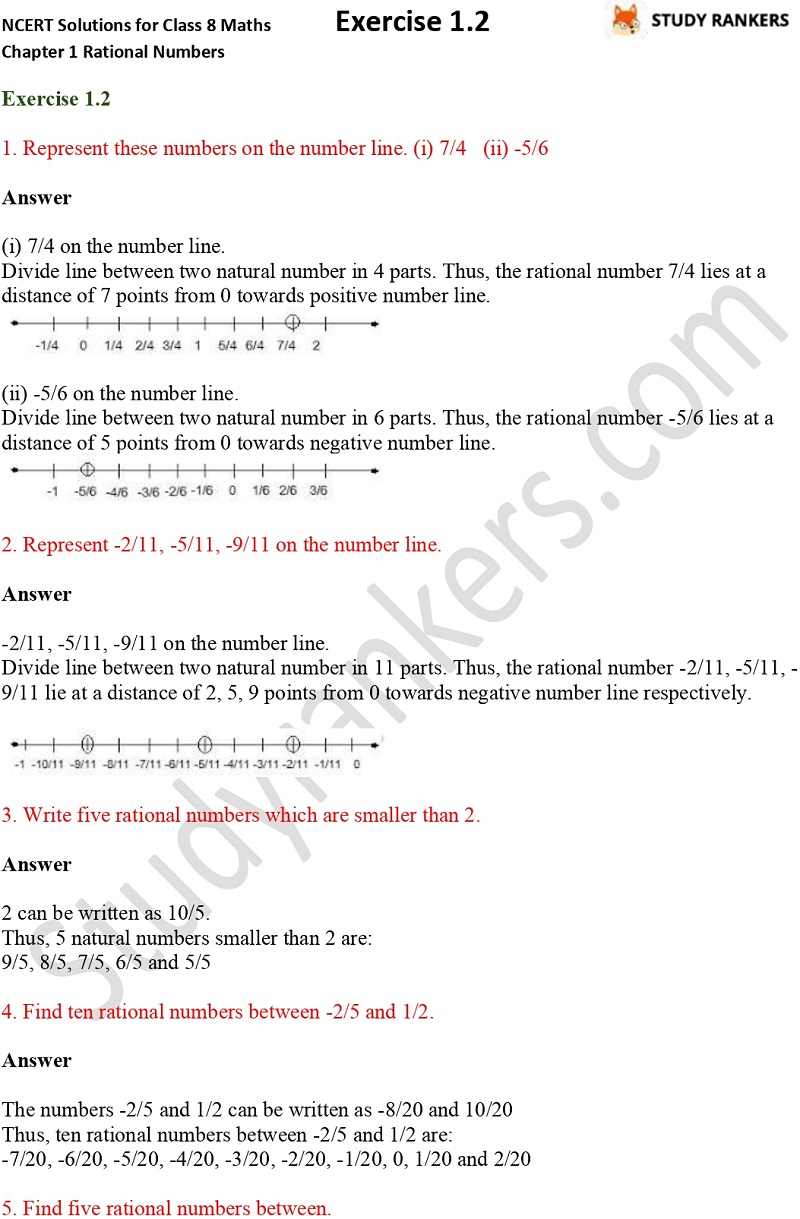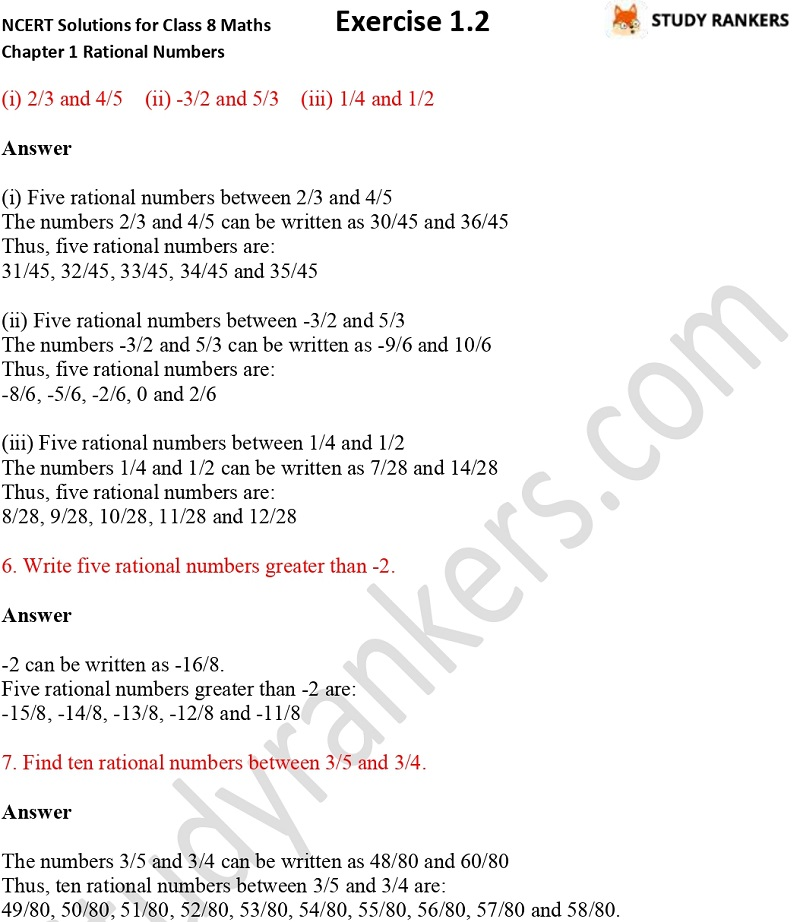>

## NCERT Solutions for Class 8 Maths Chapter 1 Rational Numbers Exercise 1.2

Chapter 1 Rational Numbers Exercise 1.2 NCERT Solutions for Class 8 Maths is provided here. These Class 8 Maths NCERT Solutions will help you in completing homework and understanding the concepts easily. By taking help of accurate and detailed questions and answers of Studyrankers, you can surely improve your marks in the examinations. These solutions are updated as per the latest syllabus of CBSE.

Exercise 1.2 has total seven questions which are about finding ten rational numbers between two given numbers, represent given number on number line etc.X